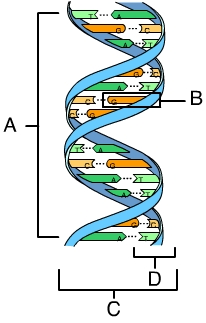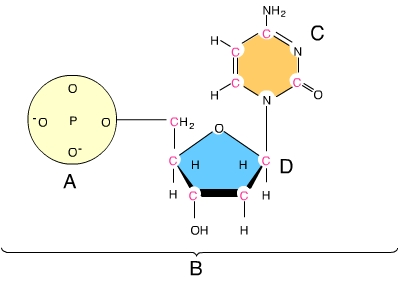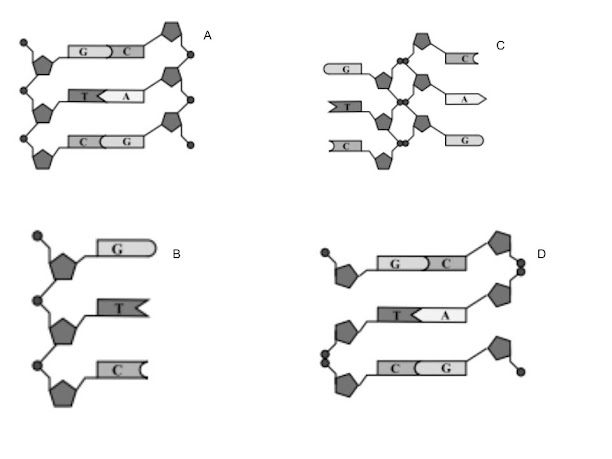Nucleic Acids MINI-Quiz
Last Name *
First Name *
Period *
1. DNA stands for
2 points
2. DNA is a long molecule (polymer) made of smaller parts called
2 points
3. An DNA nucleotide is made up of the following parts. check all that apply.
2 points
4.The diagram below (diagram 1), is often referred to as a?
2 points
Diagram 15. In the diagram below (diagram 2), which letter is pointing to a phosphate group?
2 points
Diagram 26. In a DNA double helix a Guanine of one strand always pairs with a(n) _____ of the complementary strand, and a Thymine of one strand always pairs with a(n) _____ of the complementary strand.
2 points
7. . In a sample of double-stranded DNA, 50% of the nitrogenous bases are thymine. What percentage of the nitrogenous bases in the sample are adenine?
2 points
8. Refer to Diagram 3 below to answer the following question. Which of the following models most accurately represents the structure of DNA?
2 points
Diagram 39. The primary "job" of DNA is
2 points
10. The 2 types of nucleic acids are
2 points